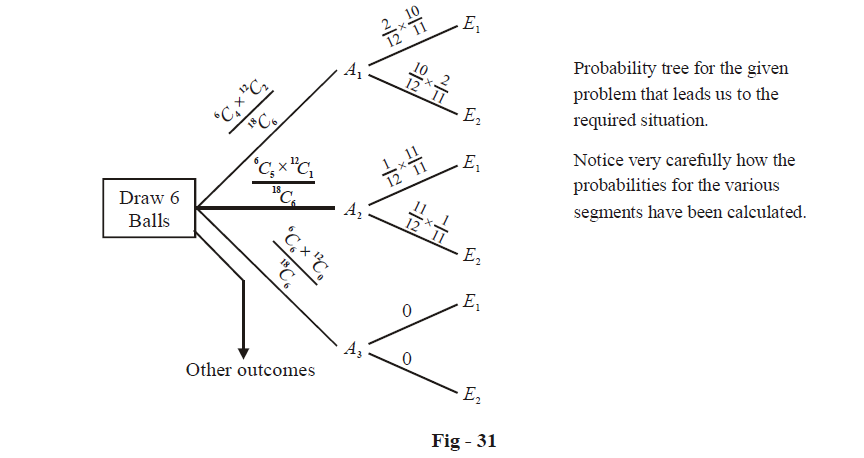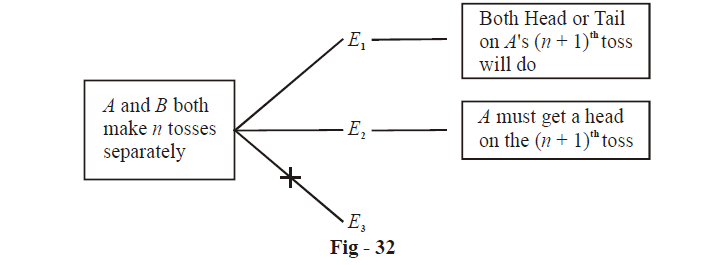# Probability Set 3

Go back to  'SOLVED EXAMPLES'

Example – 9

If 6n balls numbered 0, 1, 2, ..., 6n – 1 are placed in a bag and three are drawn at random without replacement, find the probability that the sum of the three numbers an the balls is 6n.

Solution: The important thing to realise here is that we cannot use the integral equation

${{x}_{1}}+{{x}_{2}}+{{x}_{3}}=6n\qquad.....\left( 1 \right)$

to count the number of solutions here. This is because this equation also counts those solution in which the variables might have repeated values. For example,  $${{x}_{1}}={{x}_{2}}={{x}_{3}}=2n$$  is a possible solution to (1), but it is clearly not admissible in the present case.

What we then do is count the number of solutions explicitly, using the lowest number as our ‘anchor.’

 Number on ball 1 Numbers on the other two balls Number of solutions 0 1, 6n – 1 2, 6n – 2 3, 6n – 3 $\vdots$ 3n – 1, 3n + 1 3n – 1 1 2, 6n – 3 3, 6n – 4 $\vdots$ 3n – 2, 3n + 1 3n – 1, 3n 3n – 2 2 3, 6n – 5 4, 6n – 4 $\vdots$ 3n – 2, 3n 3n – 1, 3n – 1 3n – 4 3 4, 6n – 7 5, 6n – 8 $\vdots$ 3n – 2, 3n – 1 3n – 5 $\vdots$ 2n – 1 2n  , 2n + 1 $\vdots$ 1

Go through this table thoroughly. In particular, notice carefully the terms in the last column.

We thus have the total number of favorable solutions as

\begin{align} & \left\{ (3n-1)+(3n-2) \right\}+\left\{ (3n-4)+(3n-5) \right\}+......\left\{ (5+4) \right\}+\left\{ (2+1) \right\} \\ & =\left( 6n-3 \right)+\left( 6n-9 \right)+....9+3\text{ }\left( an\text{ }A.\text{ }P. \right) \\ & =\text{ }3{{n}^{2}} \\ \end{align}

The total number of ways of choosing 3 balls out of 6n is $$^{6n}{{C}_{3}}.$$ The required probability p is therefore

\begin{align}& p=\frac{3{{n}^{2}}}{^{6n}{{C}_{3}}} \\ &\;\; =\frac{3n}{\left( 6n-1 \right)\ \left( 6n-2 \right)} \\ \end{align}

Example – 10

A bag contains 12 black and 6 white balls. 6 balls are drawn at random one by one without replacement of which at least 4 balls are white. Find the probability that in the next two draws exactly one white ball is drawn.

Solution: Let A be the event that at least 4 balls are white in the first 6 draws. Note that A can happen in three mutually exclusive ways:

A1 : exactly 4 white balls are drawn

A2 : exactly 5 white balls are drawn

A3 : exactly 6 white balls are drawn.

Now, in the next two draws, we want exactly one white ball which can happen in two ways.

E1 : First white, then black

E2 : First black, then white

Thus, we have the following probability treeNote that A has already occurred, that is, we already know that at least 4 white balls have been drawn. We want to calculate $$P\left( {{E}_{1}}|A \right)+P\left( {{E}_{2}}|A \right)$$, that is, the probability that the next two draws give exactly one white ball, given that at least 4 white balls have already been drawn in the previous 6 draws. Thus, the required probability p is :

\begin{align} & p=\frac{\underset{\text{Numerator gives the probability that }{{E}_{\mathbf{1}}}\text{ or }{{E}_{\mathbf{2}}}\text{ occur given that }A\text{ has occurred}}{\mathop{\begin{align} & P({{A}_{1}})\left\{ P({{E}_{1}}|{{A}_{1}})+P({{E}_{2}}|{{A}_{1}}) \right\}+P\left( {{A}_{2}} \right)\left\{ P({{E}_{1}}|{{A}_{2}})+P({{E}_{2}}|{{A}_{2}}) \right\} \\ & +P\left( {{A}_{3}} \right)\left\{ P({{E}_{1}}|{{A}_{3}})+P({{E}_{2}}|{{A}_{3}}) \right\} \\ \end{align}}}\,}{\underset{\text{Denominator gives the probability that }A\text{ occurs}}{\mathop{\left\{ P({{A}_{1}})+P({{A}_{2}})+P({{A}_{3}}) \right\}}}\,} \\\\ & \,\,\,=\frac{\frac{^{6}{{C}_{4}}\times {{\ }^{12}}{{C}_{2}}}{^{18}{{C}_{6}}}\ \left\{ \frac{2}{12}\times \frac{10}{11}+\frac{10}{12}\times \frac{2}{11} \right\}+\frac{^{6}{{C}_{5}}\times {{\ }^{12}}{{C}_{1}}}{^{18}{{C}_{6}}}\ \left\{ \frac{1}{12}\times \frac{11}{11}+\frac{11}{12}\times \frac{1}{11} \right\}+\frac{^{6}{{C}_{6}}\times {{\ }^{12}}{{C}_{0}}}{^{18}{{C}_{6}}}\left\{ 0+0 \right\}}{\frac{^{6}{{C}_{4}}\times {{\ }^{12}}{{C}_{2}}}{^{18}{{C}_{6}}}+\frac{^{6}{{C}_{5}}\times {{\ }^{12}}{{C}_{1}}}{^{18}{{C}_{6}}}+\frac{^{6}{{C}_{6}}\times {{\ }^{12}}{{C}_{0}}}{^{18}{{C}_{6}}}} \\\\ & \,\,\,\,=\frac{15\left( \frac{40}{132} \right)+72\left( \frac{1}{6} \right)}{15+72+1} \\\\ & \,\,\,\,=\frac{91}{484} \\ \end{align}

Example – 11

A tosses a fair coin (n + 1) times whereas B tosses a fair coin n times. Show that the probability that A gets more heads than B is  $$\frac{1}{2}$$.

Solution: Let us first consider the situation when both A and B have made n tosses.

Three mutually exclusive cases are possible for this situation:

E1 : A gets more heads than B

E2 : A and B get the same number of heads

E3 : A gets lesser heads than B

Now, for A to get a total of more heads than B, there are only two ways possible:

A1 : E1 occurs; then the result of A’s (n + 1)th toss doesn’t matter

A2 : E2 occurs; then A must get a head on the (n + 1)th toss

Thus, the probability tree for the entire scenario that leads to A getting more heads than B is:What we need to do now is calculate the probabilities P(E1) and P(E2). To do so, we define two events:

We thus have

$P({{E}_{1}})=\sum\limits_{i>j}{P({{H}_{ij}})}\ \text{and}\ P({{E}_{2}})=\sum\limits_{i=j}{P({{H}_{ij}})}$

The probability P(Hij) is straightforward to calculate, since how many heads A gets and how many B gets are independent of each other. Thus,

\begin{align} & P({{H}_{ij}})=\left( \frac{^{n}{{C}_{i}}}{{{2}^{n}}}\times \frac{^{n}{{C}_{j}}}{{{2}^{n}}} \right)\left( why? \right) \\ &\quad\qquad =\frac{^{n}{{C}_{i}}\cdot {{\ }^{n}}{{C}_{j}}}{{{2}^{2n}}} \\ \end{align}

Now,

$P({{E}_{1}})=\sum\limits_{i>j}{P({{H}_{ij}})=\frac{1}{{{2}^{2n}}}}\sum\limits_{i>j}{^{n}{{C}_{i}}}\cdot {{\ }^{n}}{{C}_{j}}$

Also,

$P({{E}_{2}})=\sum\limits_{i=j}{P({{H}_{ij}})=\frac{1}{{{2}^{2n}}}}{{\sum\limits_{i}{\left( ^{n}{{C}_{i}} \right)}}^{2}}$

Thus, our task is to calculate $$\sum\limits_{i>j}{^{n}{{C}_{i}}\cdot {{\ }^{n}}{{C}_{j}}}$$ and $${{\sum\limits_{i}{\left( ^{n}{{C}_{i}} \right)}}^{2}}$$. To do so, recall the following manipulations from Binomial theorem (appendix):

${{\left( \sum\limits_{i}{^{n}{{C}_{i}}} \right)}^{2}}={{\sum\limits_{i}{\left( ^{n}{{C}_{i}} \right)}}^{2}}+2\sum\limits_{i>j}{^{n}{{C}_{i}}\cdot {{\ }^{n}}{{C}_{j}}\,\,\,\,\,\,}...\left( 1 \right)$

and

$\sum\limits_{i=0}^{n}{{{\left( ^{n}{{C}_{i}} \right)}^{2}}}=\text{Coeff}.\ \text{of}\ {{x}^{n}}\ \text{in}\ {{(1+x)}^{2n}}={{\ }^{2n}}{{C}_{n}}\,\,\,\,\,\,...\left( 2 \right)$

Thus,

\begin{align}&\quad\;\; 2\ \sum\limits_{i=j}^{{}}{^{n}{{C}_{i}}\cdot {{\ }^{n}}{{C}_{j}}}={{\left( \sum\limits_{i}{^{n}{{C}_{i}}} \right)}^{2}}-\sum\limits_{i}{{{\left( ^{n}{{C}_{i}} \right)}^{2}}} \\ &\qquad\qquad\qquad\qquad={{2}^{2n}}-{{\ }^{2n}}{{C}_{n}}\,\left( why? \right) \\ & \Rightarrow \quad\sum\limits_{i>j}{^{n}{{C}_{i}}\cdot {{\ }^{n}}{{C}_{j}}}=\frac{{{2}^{2n}}-{{\ }^{2n}}{{C}_{n}}}{2} \\ \end{align}

Thus, we have

$P({{E}_{1}})=\frac{{{2}^{2n}}-{{\ }^{2n}}{{C}_{n}}}{{{2}^{2n+1}}}\,and\,\,P({{E}_{2}})=\frac{^{2n}{{C}_{n}}}{{{2}^{2n}}}$

Finally, from the probability tree, the required probability p is

\begin{align}& p=P({{E}_{1}})+P({{E}_{2}})\cdot \frac{1}{2} \\ & \,\,\,=\frac{{{2}^{2n}}-{{\ }^{2n}}{{C}_{n}}}{{{2}^{2n+1}}}+\frac{^{2n}{{C}_{n}}}{{{2}^{2n+1}}} \\ & \,\,\,=\frac{1}{2} \\ \end{align}

The final result is extremely simple!

Example – 12

Two numbers are selected at random without replacement from the numbers 1, 2, ....., n. Find the probability that the difference between the first and the second is not less than m where 0 < m < n.

Solution: It is clear that the total number of ways of choosing two numbers out of n is n(n – 1).

Now, let us denote by x and y the two chosen numbers, so that x represents the larger number. We need to find the number of ways in which the inequality.

$x-y\ge m$

can be satisfied.

It should be obvious that for $$x\le m$$ , no such y will exist. For x > m, to the following y will satisfy (1)

y = 1, 2, ....., xm

For example, if x = m + 4, then y can take the values 1, 2, 3, 4. Thus, we see that for (1) to be satisfied,

if some x > m is chosen, y can take on xm values from 1 to xm

Clearly, the number of ways this can be done is:

\begin{align}& \sum\limits_{x=m+1}^{n}{(x-m)}=1+2+.....+(n-m) \\ & \qquad\qquad\;\;\;\;\;\,\,\,\,\,=\frac{(n-m)\,(n-m+1)}{2} \\ \end{align}

Thus, the required probability p is

$p=\frac{(n-m)\ (n-m+1)}{2n(n-1)}$

Learn from the best math teachers and top your exams

• Live one on one classroom and doubt clearing
• Practice worksheets in and after class for conceptual clarity
• Personalized curriculum to keep up with school